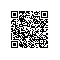# 六之再续：KMP算法之总结篇（必懂KMP）

### 引记

此前一天，一位MS的朋友邀我一起去与他讨论快速排序，红黑树，字典树，B树、后缀树，包括KMP算法，唯独在讲解KMP算法的时候，言语磕磕盼盼，我想，原因有二：1、博客内的东西不常回顾，忘了不少；2、便是我对KMP算法的理解还不够彻底，自不用说讲解自如，运用自如了。所以，特再写本篇文章。由于此前，个人已经写过关于KMP算法的两篇文章，所以，本文名为：KMP算法之总结篇。

本文分为二个部分：第一部分、再次回顾普通的BF算法与KMP算法各自的时间复杂度，并两相对照各自的匹配原理；第二部分、通过我此前一篇文章的引用，用图从头到尾详细阐述KMP算法中的next数组求法，并运用求得的next数组写出KMP算法的源码。力求让此文彻底让读者洞穿此KMP算法，所有原理，来龙去脉，让读者搞个通通透透。

在看本文之前，你心中如若对前缀和后缀这个两个概念有自己的理解，便最好了。有些东西比如此KMP算法需要我们反复思考，反复求解才行。个人写的关于KMP算法的第二篇文章为：六（续）、从KMP算法一步一步谈到BM算法ok，若有任何问题，恳请不吝指正。多谢。

### 第一部分、KMP算法初解

1普通字符串匹配BF算法与KMP算法的时间复杂度比较

KMP算法是一种线性时间复杂的字符串匹配算法，它是对BF算法（Brute-Force，最基本的字符串匹配算法的）改进。对于给的原始串S和模式串P，需要从字符串S中找到字符串P出现的位置的索引。

BF算法的时间复杂度O(strlen(S) * strlen(T))，空间复杂度O(1)

KMP算法的时间复杂度O(strlen(S) + strlen(T))，空间复杂度O(strlen(T))

2BF算法与KMP算法的区别

假设现在S串匹配到i位置，T串匹配到j位置。那么总的来说，两种算法的主要区别在于失配的情况下，对的值做的处理（注意，本文中的字符串下标都是从0开始计算）：

BF算法中，如果当前字符匹配成功，即s[i+j] == T[j]，令j++，继续匹配下一个字符；如果失配，即S[i + j] != T[j]需要让i++,并且j= 0，即每次匹配失败的情况下，模式串T相对于原始串S向右移动了一位。

而KMP算法中，如果当前字符匹配成功，即S[i]==T[j]，令i++j++，继续匹配下一个字符；如果匹配失败，即S[i] != T[j]，需要保持i不变，并且让j = next[j]，这里next[j] <=j -1，即模式串T相对于原始串S向右移动了至少1(移动的实际位数j - next[j]  >=1),

同时移动之后，i之前的部分（即S[i-j+1 ~ i-1]），和j=next[j]之前的部分（即T[0 ~ j-2]）仍然相等。显然，相对于BF算法来说，KMP移动更多的位数，起到了一个加速的作用！ (失配的特殊情形，令j=next[j]导致j==0的时候，需要将i ++，否则此时没有移动模式串)

3、BF算法为什么要回溯

i+jjT中的j++变，而动）

S：aaaacefghij

j++

T：aaac

aaaacefghij

aaac

aaaacefghij

aaac

所以，BF算法要回溯，其代码如下：

1. int Index(SString S, SString T, int pos) {
2.    //返回T在S中第pos个字符之后的位置
3.    i=pos; j=1;k=0;
4.   while ( i< = S && j< = T ) {
5.       if (S[i+k] = = T[j] ) {++k;  ++j;}   //继续比较后续字符
6.       else {i=i+1;   j=1; k=0;}      //指针回溯到 下一首位，重新开始
7.   }
8.   if(j>T) return i;          //子串结束，说明匹配成功
9.   else return  0;
10. }//Index
1. int Index(SString S, SString T, int pos) {
2. //返回T在S中第pos个字符之后的位置
3. i=pos; j=1;k=0;
4. while ( i< = S && j< = T ) {
5. if (S[i+k] = = T[j] ) {++k;  ++j;}   //继续比较后续字符
6. else {i=i+1;   j=1; k=0;}      //指针回溯到 下一首位，重新开始
7. }
8. if(j>T) return i;          //子串结束，说明匹配成功
9. else return  0;
10. }//Index

不过，也有特殊情况可以不回溯，如下：
abcdefghij(主串)
abcdefg(模式串)
即(模式串)没有相同的才不需要回溯。

4KMP 算法思想
普通的字符串匹配算法必须要回溯。但回溯就影响了效率，回溯是由T串本身的性质决定的，是因为T串本身有前后'部分匹配'的性质。像上面所说如果主串为abcdef这样的，大没有回溯的必要。

改进的地方也就是这里，我们从T串本身出发，事先就找准了T自身前后部分匹配的位置，那就可以改进算法。

如果不用回溯，那模式串下一个位置从哪里开始呢？

还是上面那个例子，T(模式串)ababc，如果c失配，那就可以往前移到aba最后一个a的位置，像这样：

...ababd...

ababc

->ababc

5、next数组的含义

令原始串为: S[i]，其中0<=i<=n；模式串为: T[j]，其中0<=j<=m

假设目前匹配到如下位置

S0,S1,S2,...,Si-j,Si-j+1...............,Si-1, Si, Si+1,....,Sn

T0,T1,...................,Tj-1, Tj, ..........

ST的绿色部分匹配成功，恰好到SiTj的时候失配，如果要保持i不变，同时达到让模式串T相对于原始串S右移的话，可以更新j的值，让Si和新的Tj进行匹配，假设新的jnext[j]表示，即让Sinext[j]匹配，显然新的j值要小于之前的j值，模式串才会是右移的效果，也就是说应该有next[j] <= j -1。那新的j值也就是next[j]应该是多少呢？我们观察如下的匹配：

1)如果模式串右移1位（从简单的思考起，移动一位会怎么样），即next[j] = j - 1， 即让蓝色的SiTj-1匹配 (注：省略号为未匹配部分)

S0,S1,S2,...,Si-j,Si-j+1...............,Si-1, Si, Si+1,....,Sn

T0,T1,...................,Tj-1, Tj, .......... (T的划线部分和S划线部分相等【1】)

T0,T1,................Tj-2,Tj-1, ....... (移动后的T的划线部分和S的划线部分相等【2】)

根据【1】【2】可以知道当next[j] =j -1，即模式串右移一位的时候，有T[0 ~ j-2] == T[1 ~ j-1]。而这两部分恰好是字符串T[0 ~j-1]的前缀和后缀，也就是说next[j]的值取决于模式串Tj前面部分的前缀和后缀相等部分的长度（好好揣摩这两个关键字概念：前缀、后缀，或者再想想，我的上一篇文章，从Trie树谈到后缀树中，后缀树的概念）。

2)如果模式串右移2位，即next[j] = j - 2， 即让蓝色的SiTj-2匹配

S0,S1,...,Si-j,Si-j+1,Si-j+2...............,Si-1, Si, Si+1,....,Sn

T0,T1,T2,...................,Tj-1, Tj, ..........(T的划线部分和S划线部分相等【3】)

T0,T1,.............,Tj-3,Tj-2,.........(移动后的T的划线部分和S的划线部分相等【4】)

同样根据【3】【4】可以知道当next[j] =j -2，即模式串右移两位的时候，有T[0 ~ j-3] == T[2 ~ j-1]。而这两部分也恰好是字符串T[0 ~j-1]的前缀和后缀，也就是说next[j]的值取决于模式串Tj前面部分的前缀和后缀相等部分的长度

3)依次类推，可以得到如下结论：当发生失配的情况下，j的新值next[j]取决于模式串中T[0 ~ j-1]中前缀和后缀相等部分的长度， 并且next[j]恰好等于这个最大长度

为此，请再允许我引用上文中的一段原文：“KMP算法中，如果当前字符匹配成功，即S[i]==T[j]，令i++j++，继续匹配下一个字符；如果匹配失败，即S[i] != T[j]，需要保持i不变，并且让j = next[j]，这里next[j] <=j -1，即模式串T相对于原始串S向右移动了至少1(移动的实际位数j - next[j]  >=1),

同时移动之后，i之前的部分（即S[i-j+1 ~ i-1]），和j=next[j]之前的部分（即T[0 ~ j-2]）仍然相等。显然，相对于BF算法来说，KMP移动更多的位数，起到了一个加速的作用！ (失配的特殊情形，令j=next[j]导致j==0的时候，需要将i ++，否则此时没有移动模式串)。”

于此，也就不难理解了我的关于KMP算法的第二篇文章之中：“当匹配到S[i] != P[j]的时候有 S[i-j…i-1] = P[0…j-1]. 如果下面用j_next去匹配，则有P[0…j_next-1] = S[i-j_next…i-1] = P[j-j_next…j-1]。此过程如下图3-1所示。

当匹配到S[i] != P[j]时，S[i-j…i-1] = P[0…j-1]

S: 0 … i-j … i-1 i …

P:       0 …   j-1 j …

如果下面用j_next去匹配，则有P[0…j_next-1] = S[i-j_next…i-1] = P[j-j_next…j-1]。

P: 0 … j-j_next  .…j-1_    …

P:        0    … .j_next-1 …

所以，根据上面两个步骤，推出下一匹配位置j_next:

S: 0 … i-j … i-j_next …   i-1      i …

P:                   0   … j_next-1  j_next …

图3-1 求j-next（最大的值）的三个步骤

下面，我们用变量k来代表求得的j_next的最大值，即k表示这S[i]、P[j]不匹配时P中下一个用来匹配的位置，使得P[0…k-1] = P[j-k…j-1]，而我们要尽量找到这个k的最大值。”。

根据上文的【1】与【2】的匹配情况，可得第二篇文章之中所谓的k=1，根据上文的【3】与【4】的匹配情况，k=2。

所以，归根究底，KMP算法的本质便是：针对待匹配的模式串的特点，判断它是否有重复的字符，从而找到它的前缀与后缀，进而求出相应的Next数组，最终根据Next数组而进行KMP匹配。

6、Next数组的具体求法

上面给出了next数组的含义，下面给出求这个数组的具体算法。

1）显然有next = 0,next = 0

2）观察【1】【2】可以看到如果T[j]==T[j -1]T[j] == T[next[j]]的情况下(一定要注意 next[j]小，有助于理清思路)j+1前面字符串的前缀和后缀的相等部分长度增加了1，所以有T[j]==T[next[j]]的时候，next[j+1] = next[j ] + 1;

同样观察【3】【4】也可以看到如果T[j]==T[j-2]亦即T[j]==T[next[j]的情况下，j+1前面的字符串的前缀和后缀相等部分的长度增加了1，所以也有T[j]==T[next[j]]的时候，next[j+1] = next[j] + 1;

综合上面的规律有当T[j] == T[next[j]]的情况下next[j+1]=next[j] + 1;

3) T[j] != T[next[j]]的情况next[j+1]又该等于多少呢？拿【1】【2】来说，如果此时T[j] != T[j-1]，可以移动【2】对应的串，直到【1】中的Tj等于下面【2】中对应的字符，此时就找到了j+1的最大前后缀。

注意，移动的时候同样可以用到已经计算出的next数组的值。接下来，进入本文的第二部分。

### 第二部分、next数组求法的来龙去脉与KMP算法的源码

本部分引自个人此前的关于KMP算法的第二篇文章：六之续、由KMP算法谈到BM算法。前面，我们已经知道即不能让P[j]=P[next[j]]成立成立。不能再出现上面那样的情况啊！即不能有这种情况出现：P=b，而竟也有P[next]=P=b

正如在第二篇文章中，所提到的那样：“这里读者理解可能有困难的是因为文中，时而next，时而nextval，把他们的思维搞混乱了。其实next用于表达数组索引，而nextval专用于表达next数组索引下的具体各值，区别细微。至于文中说不允许P=P[next[j] ]出现，是因为已经有P=b与S匹配败，而P[next]=P1=b，若再拿P=b去与S匹配则必败。”--六之续、由KMP算法谈到BM算法。

又恰恰如上文中所述：“模式串T相对于原始串S向右移动了至少1(移动的实际位数j - next[j]  >=1)

ok，求next数组的get_nextval函数正确代码如下：

1. //代码4-1
2. //修正后的求next数组各值的函数代码
3. void get_nextval(char const* ptrn, int plen, int* nextval)
4. {
5.     int i = 0;
6.     nextval[i] = -1;
7.     int j = -1;
8.     while( i < plen-1 )
9.     {
10.         if( j == -1 || ptrn[i] == ptrn[j] )   //循环的if部分
11.         {
12.             ++i;
13.             ++j;
14.             //修正的地方就发生下面这4行
15.             if( ptrn[i] != ptrn[j] ) //++i，++j之后，再次判断ptrn[i]与ptrn[j]的关系
16.                 nextval[i] = j;      //之前的错误解法就在于整个判断只有这一句。
17.             else
18.                 nextval[i] = nextval[j];
19.         }
20.         else                                 //循环的else部分
21.             j = nextval[j];
22.     }
23. }
1. //代码4-1
2. //修正后的求next数组各值的函数代码
3. void get_nextval(char const* ptrn, int plen, int* nextval)
4. {
5. int i = 0;
6. nextval[i] = -1;
7. int j = -1;
8. while( i < plen-1 )
9. {
10. if( j == -1 || ptrn[i] == ptrn[j] )   //循环的if部分
11. {
12. ++i;
13. ++j;
14. //修正的地方就发生下面这4行
15. if( ptrn[i] != ptrn[j] ) //++i，++j之后，再次判断ptrn[i]与ptrn[j]的关系
16. nextval[i] = j;      //之前的错误解法就在于整个判断只有这一句。
17. else
18. nextval[i] = nextval[j];
19. }
20. else                                 //循环的else部分
21. j = nextval[j];
22. }
23. }

举个例子，举例说明下上述求next数组的方法。
S a b a b a b c
P a b a b c
S != P
那么下一个和S匹配的位置是k=2(也即P[next])。此处的k=2也再次佐证了上文第3节开头处关于为了找到下一个匹配的位置时k的求法。上面的主串与模式串开头4个字符都是“abab”，所以，匹配失效后下一个匹配的位置直接跳两步继续进行匹配。
S a b a b a b c
P      a b a b c

P的next数组值分别为-1 0 -1 0 2

next数组各值怎么求出来的呢?分以下五步：

1. 初始化：i=0，j=-1，由于j == -1，进入上述循环的if部分，++i得i=1，++j得j=0，且ptrn[i] != ptrn[j]（即a！=b）），所以得到第二个next值即nextval = 0；
2. i=1，j=0，进入循环esle部分，j=nextval[j]=nextval=-1；
3. 进入循环的if部分，++i，++j，i=2，j=0，因为ptrn[i]=ptrn[j]=a,所以nextval=nextval=-1；
4. i=2, j=0, 由于ptrn[i]=ptrn[j],再次进入循环if部分，所以++i=3，++j=1,因为ptrn[i]=ptrn[j]=b,所以nextval=nextval=0；
5. i=3,j=1,由于ptrn[i]=ptrn[j]=b,所以++i=4，++j=2,退出循环。

这样上例中模式串的next数组各值最终应该为:图4-1 正确的next数组各值
next数组求解的具体过程如下：
初始化：nextval = -1，我们得到第一个next值即-1.图4-2 第一个next值即-1

i = 0，j = -1，由于j == -1，进入上述循环的if部分，++i得i=1，++j得j=0，且ptrn[i] != ptrn[j]（即a！=b）），所以得到第二个next值即nextval = 0；图4-3 第二个next值0

上面我们已经得到，i= 1，j = 0，由于不满足条件j == -1 || ptrn[i] == ptrn[j]，所以进入循环的esle部分，得j = nextval[j] = -1；此时，仍满足循环条件，由于i = 1，j = -1，因为j == -1，再次进入循环的if部分，++i得i=2，++j得j=0，由于ptrn[i] == ptrn[j]（即ptrn=ptrn，也就是说第1个元素和第三个元素都是a），所以进入循环if部分内嵌的else部分，得到nextval = nextval = -1；图4-4 第三个next数组元素值-1

i = 2，j = 0，由于ptrn[i] == ptrn[j]，进入if部分，++i得i=3，++j得j=1，所以ptrn[i] == ptrn[j]（ptrn==ptrn，也就是说第2个元素和第4个元素都是b），所以进入循环if部分内嵌的else部分，得到nextval = nextval = 0；图4-5 第四个数组元素值0
如果你还是没有弄懂上述过程是怎么一回事，请现在拿出一张纸和一支笔出来，一步一步的画下上述过程。相信我，把图画出来了之后，你一定能明白它的。
然后，我留一个问题给读者，为什么上述的next数组要那么求?有什么原理么?

提示：我们从上述字符串abab 各字符的next值-1 0 -1 0，可以看出来，根据求得的next数组值，偷用前缀、后缀的概念，一定可以判断出在abab之中，前缀和后缀相同，即都是ab，反过来，如果一个字符串的前缀和后缀相同，那么根据前缀和后缀依次求得的next各值也是相同的。

• 5、利用求得的next数组各值运用Kmp算法

Ok，next数组各值已经求得，万事俱备，东风也不欠了。接下来，咱们就要应用求得的next值，应用KMP算法来匹配字符串了。还记得KMP算法是怎么一回事吗?容我再次引用下之前的KMP算法的代码，如下：

1. //代码5-1
2. //int kmp_seach(char const*, int, char const*, int, int const*, int pos)  KMP模式匹配函数
3. //输入：src, slen主串
4. //输入：patn, plen模式串
5. //输入：nextval KMP算法中的next函数值数组
6. int kmp_search(char const* src, int slen, char const* patn, int plen, int const* nextval, int pos)
7. {
8.     int i = pos;
9.     int j = 0;
10.     while ( i < slen && j < plen )
11.     {
12.         if( j == -1 || src[i] == patn[j] )
13.         {
14.             ++i;
15.             ++j;
16.         }
17.         else
18.         {
19.             j = nextval[j];
20.             //当匹配失败的时候直接用p[j_next]与s[i]比较，
21.             //下面阐述怎么求这个值，即匹配失效后下一次匹配的位置
22.         }
23.     }
24.     if( j >= plen )
25.         return i-plen;
26.     else
27.         return -1;
28. }
1. //代码5-1
2. //int kmp_seach(char const*, int, char const*, int, int const*, int pos)  KMP模式匹配函数
3. //输入：src, slen主串
4. //输入：patn, plen模式串
5. //输入：nextval KMP算法中的next函数值数组
6. int kmp_search(char const* src, int slen, char const* patn, int plen, int const* nextval, int pos)
7. {
8. int i = pos;
9. int j = 0;
10. while ( i < slen && j < plen )
11. {
12. if( j == -1 || src[i] == patn[j] )
13. {
14. ++i;
15. ++j;
16. }
17. else
18. {
19. j = nextval[j];
20. //当匹配失败的时候直接用p[j_next]与s[i]比较，
21. //下面阐述怎么求这个值，即匹配失效后下一次匹配的位置
22. }
23. }
24. if( j >= plen )
25. return i-plen;
26. else
27. return -1;
28. }图5-1 求得的正确的next数组元素各值

以下是匹配过程，分三步：
第一步：主串和模式串如下，S与P匹配失败。图5-2 第一步，S与P匹配失败
第二步：S保持不变，P的下一个匹配位置是P[next]，而next=0,所以P[next]=P，即P与S匹配。在P与S处匹配失败。图5-3 第二步，在P与S处匹配失败

第三步：与上文中第3小节末的情况一致。由于上述第三步中，P与S还是不匹配。此时i=3,j=nextval=-1,由于满足条件j==-1，所以进入循环的if部分,++i=4,++j=0,即主串指针下移一个位置，从P与S处开始匹配。最后j==plen，跳出循环，输出结果i-plen=4(即字串第一次出现的位置），匹配成功，算法结束。图5-4 第三步，匹配成功，算法结束
所以，综上，总结上述三步为：

1. 开始匹配，直到P！=S，匹配失败；
2. nextval=0，所以P继续与S匹配，再次匹配失败；
3. nextval=-1，满足循环if部分条件j==-1，所以，++i，++j，主串指针下移一个位置，从P与S处开始匹配，最后j==plen，跳出循环，输出结果i-plen=4，算法结束。

### 后记

OK， 相信，看过此文后，无论是谁，都一定可以把KMP算法搞懂了（但万一还是有读者没有搞懂，那怎么办呢？还有最后一个办法：把本文打印下来，再仔细琢磨。如果是真真正正想彻底弄懂某一个东西，那么必须付出些代价。但万一要是打印下来了却还是没有弄懂呢？那来北京找我吧，我手把手教你。祝好运）。谢谢，完。
July、二零一一年十二月五日中午。使用钉钉扫一扫加入圈子
+ 订阅